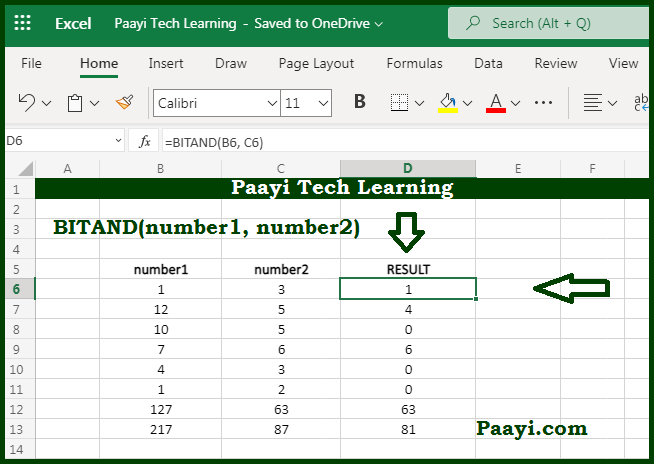# Learn How to Use Microsoft Excel BITAND Function

Written by | 0 Comments | 580 Views

In this article, you will learn how to use the Microsoft Excel BITAND function and its prime function in Microsoft Excel. You will also get to know the Microsoft Excel BITAND function return value and syntax with the help of some examples.

Microsoft Excel BITAND Function

The main function of Microsoft Excel BITAND function is used to return the "Bitwise And" of two numbers. That implies with the help of BITAND function; you can able to return the decimal number representing the bitwise AND of two numbers.

Return Value of BITAND Function

The return value will be the decimal number.

Syntax of BITAND Function

=BITAND(number1, number2)

Where the argument:

number1: This is the positive decimal number.

number2: This is the positive decimal number.

## How to Use Microsoft Excel BITAND Function?The input number can be greater than or equal to zero and no longer than  2^48 - 1). The BITAND function performs logical AND operation on each corresponding bit in the binary representation of the two numbers.